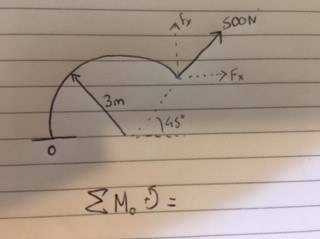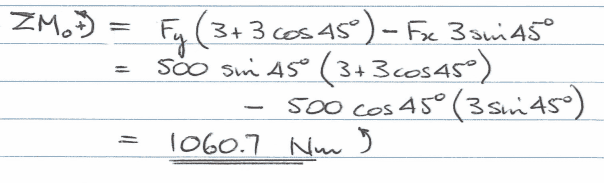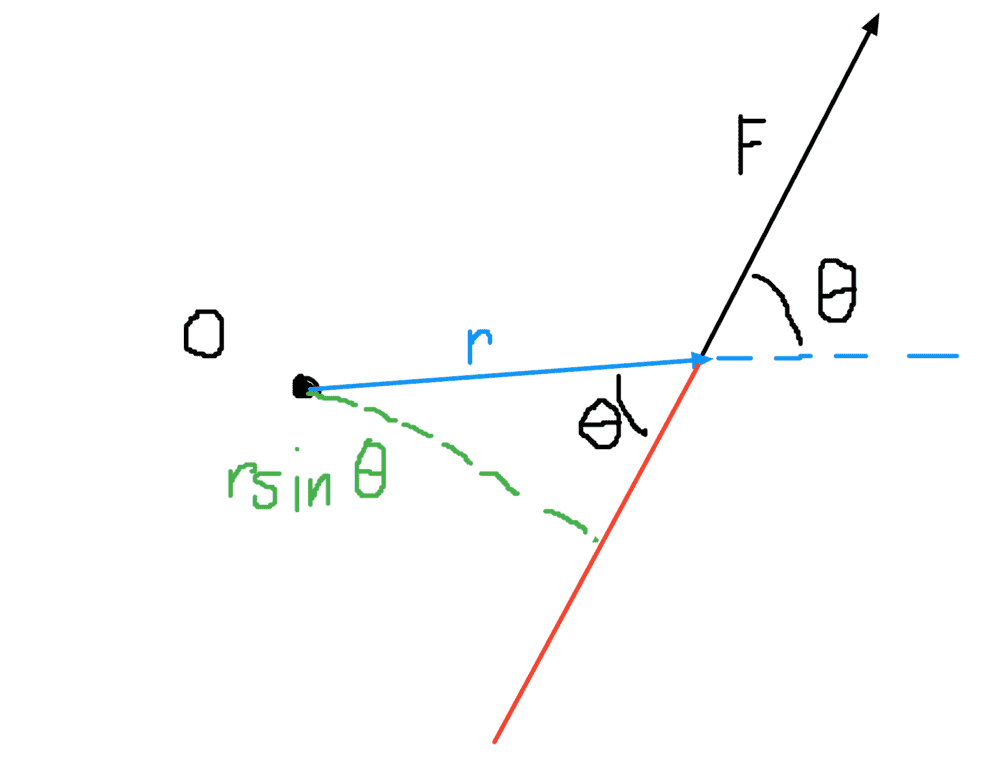# Moment of a force about point O

• Engineering
Homework Statement:
Determine the moment of the force around point O
Relevant Equations:
M=fd
G'day.
I have a problem with my statics work, i understand its probably quite simple but im having trouble in my understanding.
Essentially problems make more sense to me by using the component method, although in saying that i understand this problem would be quite a lot more simple were i to approach it without the component method.heres the vector diagram for the problem,this is the answer for the sum of the components, but im confused why Fx is negative and why Fy is being multiplied by 3+3cos45, i understand the barebones equation for the moment is M=fd(perpendicular), but from my understanding Fx would be acting in the positive (right) direction.

mfb
Mentor
Fx as drawn in the diagram pulls clockwise, i.e. in negative direction as angles are counterclockwise by definition.

3+3cos(45 deg) is the horizontal distance between O and the point where the force acts.

this problem would be quite a lot more simple were i to approach it without the component method.

You can do it without components by remembering the fact that we can find the moment by multiplying the force by the perpendicular distance to the line of action of the force?

What does 'line of action mean'? That is the extension of the force vector in both directions to form a line - it shows the direction along which the force acts. I hope that makes sense

Why is this the case?
$$\vec M = \vec r \times \vec F = |F||r|sin(\theta) \hat n$$
(EDIT: the ## \hat n ## denotes the direction of the moment vector as explained below by @etotheipi ) where we can interpret ## r sin(\theta) ## as the perpendicular distance (from the origin) to the extended line of action. Just in case this doesn't make sense I have included an illustration below. Apologies I am not used to writing with my mouse. The red line is an extension of the force vector and represents the 'line of action'. As we can see the force is ## F ## and the perpendicular distance to the line of action is ## r sin(\theta) ## and therefore the magnitude of the moment is ##Frsin(\theta) ##Now back to the main problem, that means that we don't need to use components if we can find the perpendicular distance to the line of action of the force (we already know what F is) and then we can multiply the two. I would suggest that you extend the force vector line below point ## O ##.

Complete the semicircle that touches the line of action and see whether that helps

If this still doesn't make sense, then I will be happy to clarify where possible.

Last edited:
•etotheipi
etotheipi
$$M = \vec r \times \vec F = |F||r|sin(\theta)$$

I would just clarify that the moment is a vector orthogonal to ##\vec{r}## and ##\vec{F}##,$$\vec{M} = \vec{r} \times \vec{F} = |\vec{F}| |\vec{r}| \sin{(\theta)} \hat{n}$$The component along the ##\hat{n}## direction is$$M_n = (\vec{r} \times \vec{F})_n = |\vec{F}| |\vec{r}|\sin{(\theta)}$$ And its magnitude is$$|\vec{M}| = |\vec{r} \times \vec{F}| = |\vec{F}| |\vec{r}| |\sin{(\theta)}|$$

•Master1022
Lnewqban
Gold Member
Homework Statement:: Determine the moment of the force around point O
Relevant Equations:: M=fd

... but im confused why Fx is negative and why Fy is being multiplied by 3+3cos45, i understand the barebones equation for the moment is M=fd(perpendicular), but from my understanding Fx would be acting in the positive (right) direction.

Welcome, Jason234578In addition to the excelent explanations above:
In moment problems like this one, once you can see the position of each component of force respect to the common fulcrum, it is easy to visually determine the sign of the individual moment that each of those components tend to induce.

In order to do the summation of the individual moments, by convention we consider counter-clockwise "rotations" to be positive and clockwise "rotations" to be negative.

In your specific problem, Fx alone would tend to rotate the body, part or structure clockwise, reason for which moment induced by Fx has that negative sign in the summation equation, even when the direction of Fx itself is positive, as you have properly noted.

The rest is only application of geometry and trigonometry, trying to find the horizontal and vertical distances (as the force components are only horizontal and vertical as well) between the line of action of each force component and the common fulcrum.

•sysprog